# 30 OOPs Interview Questions and Answers (2023)

Object-Oriented Programming, or OOPs, is a programming paradigm that implements the concept of objects in the program. It aims to provide an easier solution to real-world problems by implementing real-world entities such as inheritance, abstraction, polymorphism, etc. in programming. OOPs concept is widely used in many popular languages like Java, Python, C++, etc.OOPs is also one of the most important topics for programming interviews. This article contains some top interview questions on the OOPs concept.

## OOPs Interview Questions

### 1. What is Object Oriented Programming (OOPs)?

Object Oriented Programming (also known as OOPs) is a programming paradigm where the complete software operates as a bunch of objects talking to each other. An object is a collection of data and the methods which operate on that data.

### 2. Why OOPs?

The main advantage of OOP is better manageable code that covers the following:

1. The overall understanding of the software is increased as the distance between the language spoken by developers and that spoken by users.
2. Object orientation eases maintenance by the use of encapsulation.  One can easily change the underlying representation by keeping the methods the same.
3. The OOPs paradigm is mainly useful for relatively big software.

### 3. What is a Class?

A class is a building block of Object Oriented Programs. It is a user-defined data type that contains the data members and member functions that operate on the data members. It is like a blueprint or template of objects having common properties and methods.

### 4. What is an Object?

An object is an instance of a class. Data members and methods of a class cannot be used directly. We need to create an object (or instance) of the class to use them. In simple terms, they are the actual world entities that have a state and behavior.

Eg. The code below shows is an example of how an instance of a class (i.e an object ) of a class is created

## C++

 `#include ` `using` `namespace` `std; ` ` `  `class` `Student{ ` `  ``private``: ` `    ``string name; ` `    ``string surname; ` `    ``int` `rollNo; ` ` `  `  ``public``: ` `    ``Student(string studentName, string studentSurname, ``int` `studentRollNo){ ` `        ``name = studentName; ` `        ``surname = studentSurname; ` `        ``rollNo = studentRollNo; ` `    ``} ` ` `  `    ``void` `getStudentDetails(){ ` `        ``cout << ``"The name of the student is "` `<< name << ``" "` `<< surname << endl; ` `        ``cout << ``"The roll no of the student is "` `<< rollNo << endl; ` `    ``} ` `}; ` ` `  `int` `main() { ` `    ``Student student1(``"Vivek"``, ``"Yadav"``, 20); ` `    ``student1.getStudentDetails(); ` ` `  `    ``return` `0; ` `} `

## Java

 `class` `Student{ ` `  ``String name; ` `  ``String surname; ` `  ``int` `rollNo; ` `  ``Student(String studentName, String studentSurname, ``int` `studentRollNo){ ` `    ``this``.name= studentName; ` `    ``this``.surname = studentSurname; ` `    ``this``.rollNo= studentRollNo; ` `  ``} ` `  ``public` `void` `getStudentDetails(){ ` `    ``System.out.println(``"The name of the student is "``+ ``this``.name +``" "``+ ``this``.surname); ` `    ``System.out.println(``"The roll no of the student is "``+ ``this``.rollNo); ` `  ``} ` `} ` `class` `OOPS{ ` `   ``public` `static` `void` `main(String args[]) { ` `       ``Student student1 = ``new` `Student(``"Vivek"``, ``"Yadav"` `, ``20``); ` `       ``student1.getStudentDetails(); ` `   ``} ` `}`

## Python3

 `# code ` `class` `Student: ` `    ``def` `__init__(``self``, studentName, studentSurname, studentRollNo): ` `        ``self``.name ``=` `studentName ` `        ``self``.surname ``=` `studentSurname ` `        ``self``.rollNo ``=` `studentRollNo ` ` `  `    ``def` `getStudentDetails(``self``): ` `        ``print``(``"The name of the student is"``, ``self``.name, ``self``.surname) ` `        ``print``(``"The roll no of the student is"``, ``self``.rollNo) ` ` `  ` `  `student1 ``=` `Student(``"Vivek"``, ``"Yadav"``, ``20``) ` `student1.getStudentDetails() `

## C#

 `using` `System; ` ` `  `public` `class` `Student { ` `    ``private` `string` `name; ` `    ``private` `string` `surname; ` `    ``private` `int` `rollNo; ` ` `  `    ``public` `Student(``string` `studentName, ``string` `studentSurname, ``int` `studentRollNo) { ` `        ``name = studentName; ` `        ``surname = studentSurname; ` `        ``rollNo = studentRollNo; ` `    ``} ` ` `  `    ``public` `void` `GetStudentDetails() { ` `        ``Console.WriteLine(``"The name of the student is {0} {1}"``, name, surname); ` `        ``Console.WriteLine(``"The roll no of the student is {0}"``, rollNo); ` `    ``} ` `} ` ` `  `class` `Program { ` `    ``static` `void` `Main(``string``[] args) { ` `        ``Student student1 = ``new` `Student(``"Vivek"``, ``"Yadav"``, 20); ` `        ``student1.GetStudentDetails(); ` ` `  `        ``Console.ReadKey(); ` `    ``} ` `} `

Output

```The name of the student is Vivek Yadav
The roll no of the student is 20```

### 5. What are the main features of OOPs?

The main feature of the OOPs, also known as 4 pillars or basic principles of OOPs are as follows:

1. Encapsulation
2. Data Abstraction
3. Polymorphism
4. Inheritance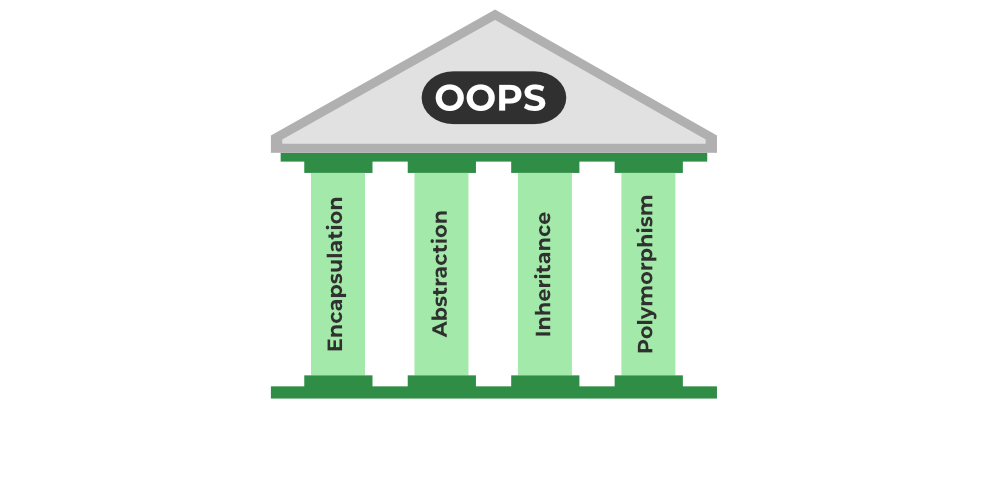OOPs Main Features

### 6. What is Encapsulation?

Encapsulation is the binding of data and methods that manipulate them into a single unit such that the sensitive data is hidden from the users
It is implemented as the processes mentioned below:

1. Data hiding: A language feature to restrict access to members of an object. For example, private and protected members in C++.
2. Bundling of data and methods together: Data and methods that operate on that data are bundled together. For example, the data members and member methods that operate on them are wrapped into a single unit known as a class.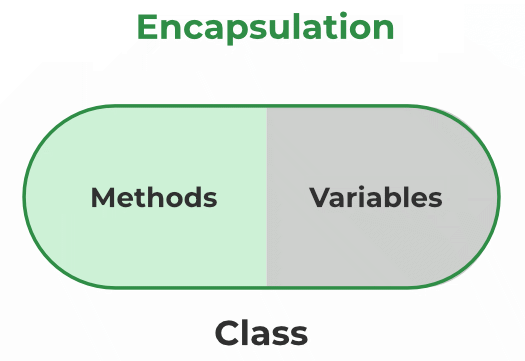## Java

 `//simple demonstration of encapsulation ` `//It has a private data member and getter and setter methods.   ` `public` `class` `Student{   ` `    ``//private data members   ` `    ``private` `String name;   ` `      ``private` `int` `rollNo; ` `    ``//public getter method to access the name   ` `    ``public` `String getName(){   ` `        ``return` `name;   ` `    ``}   ` `  ``//public getter method to access rollNo   ` `    ``public` `int` `getRollNo(){   ` `        ``return` `rollNo;   ` `    ``}  ` `//public setter method to set name   ` `    ``public` `void` `setName(String name){   ` `            ``this``.name=name   ` `    ``}   ` `  ``//public setter method to set rollNo   ` `    ``public` `void` `setRollNo(``int` `rollNo){   ` `            ``this``.rollNo=rollNo;   ` `    ``}   ` `}`

### 7. What is Abstraction?

Abstraction is similar to data encapsulation and is very important in OOP. It means showing only the necessary information and hiding the other irrelevant information from the user. Abstraction is implemented using classes and interfaces.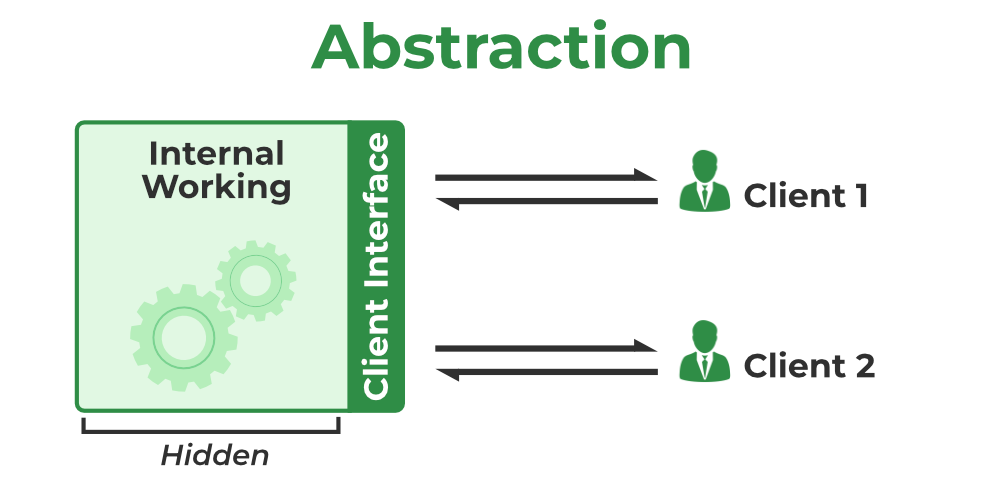## Java

 `//implementation of abstraction through abstract class ` `abstract` `class` `Animal { ` `    ``abstract` `void` `walk(); ` `    ``void` `eat() ` `    ``{ ` `        ``System.out.println(``"The animal is eating."``); ` `    ``} ` `    ``Animal() ` `    ``{ ` `        ``System.out.println( ` `            ``"An Animal is going to be created."``); ` `    ``} ` `} ` ` `  `class` `Cow ``extends` `Animal { ` `    ``Cow() { System.out.println(``"You have created a Cow"``); } ` `    ``void` `walk() { System.out.println(``"Cow is walking."``); } ` `} ` ` `  `class` `Goat ``extends` `Animal { ` `    ``Goat() ` `    ``{ ` `        ``System.out.println(``"You have created a Goat"``); ` `    ``} ` `    ``void` `walk() { System.out.println(``"Goat is walking."``); } ` `} ` ` `  `public` `class` `OOPS { ` `    ``public` `static` `void` `main(String args[]) ` `    ``{ ` `        ``Cow cow = ``new` `Cow(); ` `        ``cow.walk(); ` `        ``cow.eat(); ` `        ``Goat goat = ``new` `Goat(); ` `        ``goat.walk(); ` `        ``goat.eat(); ` `    ``} ` `}`

### 8. What is Polymorphism?

The word “Polymorphism” means having many forms. It is the property of some code to behave differently for different contexts. For example, in C++ language, we can define multiple functions having the same name but different working depending on the context.

Polymorphism can be classified into two types based on the time when the call to the object or function is resolved. They are as follows:

A. Compile Time Polymorphism
B. Runtime Polymorphism

A) Compile-Time Polymorphism

Compile time polymorphism, also known as static polymorphism or early binding is the type of polymorphism where the binding of the call to its code is done at the compile time. Method overloading or operator overloading are examples of compile-time polymorphism.

B) Runtime Polymorphism

Also known as dynamic polymorphism or late binding, runtime polymorphism is the type of polymorphism where the actual implementation of the function is determined during the runtime or execution. Method overriding is an example of this method.

## Java

 `// An example of method overloading ` `class` `Student { ` `   ``String name,surname; ` `   ``int` `rollNo; ` `   `  `   ``public` `void` `showStudentDetails(String name) { ` `       ``System.out.println(``"The name of the student is "` `+ name); ` `   ``} ` ` `  ` `  `   ``public` `void` `showStudentDetails(``int` `rollNo) { ` `       ``System.out.println(``"the roll no of the student is "``+ rollNo); ` `   ``} ` ` `  ` `  `   ``public` `void` `showStudentDetails(String name, String surname, ``int` `rollNo) { ` `       ``System.out.println(name); ` `       ``System.out.println(surname); ` `       ``System.out.println(age); ` `   ``} ` `} `

## Java

 `// an example of method oerriding ` `class` `Student { ` `   ``public` `void` `read() { ` `       ``System.out.println(``"The student is reading"``); ` `   ``} ` `} ` `class` `SchoolStudent ``extends` `Student { ` `   ``public` `void` `read(String book) { ` `       ``System.out.println(``"the student is reding "``+ book); ` `   ``}   ` `} ` `class` `CollegeStudent ``extends` `Student { ` `   ``public` `void` `read(String researchPaper , String labJournal) { ` `       ``System.out.println(``"the student is reading "``+researchPaper +``" and "``+ labJournal); ` `   ``}   ` `} `

### 9. What is Inheritance? What is its purpose?

The idea of inheritance is simple, a class is derived from another class and uses data and implementation of that other class. The class which is derived is called child or derived or subclass and the class from which the child class is derived is called parent or base or superclass.

The main purpose of Inheritance is to increase code reusability. It is also used to achieve Runtime Polymorphism.

## Java

 `// an example of inheritance ` `class` `Student { ` `   ``public` `void` `read() { ` `       ``System.out.println(``"The student is reading"``); ` `   ``} ` `} ` `class` `SchoolStudent ``extends` `Student { ` `   ``public` `void` `read(String book) { ` `       ``System.out.println(``"the student is reding "``+ book); ` `   ``}   ` `}`

### 10. What are access specifiers? What is their significance in OOPs?

Access specifiers are special types of keywords that are used to specify or control the accessibility of entities like classes, methods, and so on. Private, Public, and Protected are examples of access specifiers or access modifiers.
The key components of OOPs, encapsulation and data hiding, are largely achieved because of these access specifiers.

## Java

 `class` `User { ` `   ``public` `String userName; ` `   ``protected` `String userEmail; ` `   ``private` `String password; ` ` `  ` `  `   ``public` `void` `setPassword(String password) { ` `       ``this``.password = password; ` `   ``} ` `} ` `public` `class` `OOPS { ` `   ``public` `static` `void` `main(String args[]) { ` `       ``User user1 = ``new` `User(); ` `       ``user1.userName = ``"Vivek_Kumar_Yadav"``; ` `       ``user1.setPassword(``"abcd@12345"``); ` `       ``user1.userEmail = ``"abc@gmail.com"``; ` `   ``} ` `} `

### 12. What other paradigms of programming exist besides OOPs?

The programming paradigm is referred to the technique or approach of writing a program. The programming paradigms can be classified into the following types: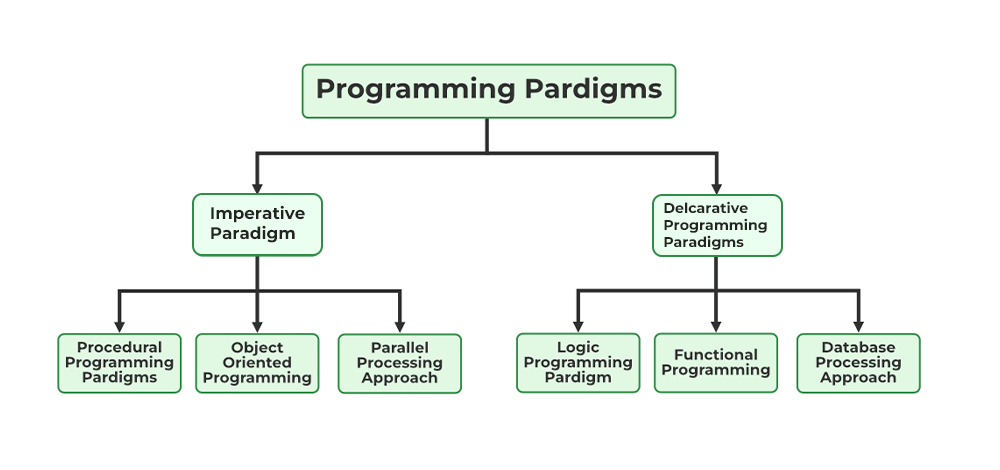It is a programming paradigm that works by changing the program state through assignment statements. The main focus in this paradigm is on how to achieve the goal. The following programming paradigms come under this category:

1. Procedural Programming Paradigm: This programming paradigm is based on the procedure call concept. Procedures, also known as routines or functions are the basic building blocks of a program in this paradigm.
2. Object-Oriented Programming or OOP: In this paradigm, we visualize every entity as an object and try to structure the program based on the state and behavior of that object.
3. Parallel Programming: The parallel programming paradigm is the processing of instructions by dividing them into multiple smaller parts and executing them concurrently.

Declarative programming focuses on what is to be executed rather than how it should be executed. In this paradigm, we express the logic of a computation without considering its control flow. The declarative paradigm can be further classified into:

1. Logical Programming Paradigm: It is based on formal logic where the program statements express the facts and rules about the problem in the logical form.
2. Functional Programming Paradigm: Programs are created by applying and composing functions in this paradigm.
3. Database Programming Paradigm: To manage data and information organized as fields, records, and files, database programming models are utilized.

### 13. What is the difference between Structured Programming and Object Oriented Programming?

Structured Programming is a technique that is considered a precursor to OOP and usually consists of well-structured and separated modules. It is a subset of procedural programming. The difference between OOPs and Structured Programming is as follows:

### 14. What are some commonly used Object Oriented Programming Languages?

OOPs paradigm is one of the most popular programming paradigms. It is widely used in many popular programming languages such as:

### 15. What are the different types of Polymorphism?

Polymorphism can be classified into two types based on the time when the call to the object or function is resolved. They are as follows:

1. Compile Time Polymorphism
2. Runtime Polymorphism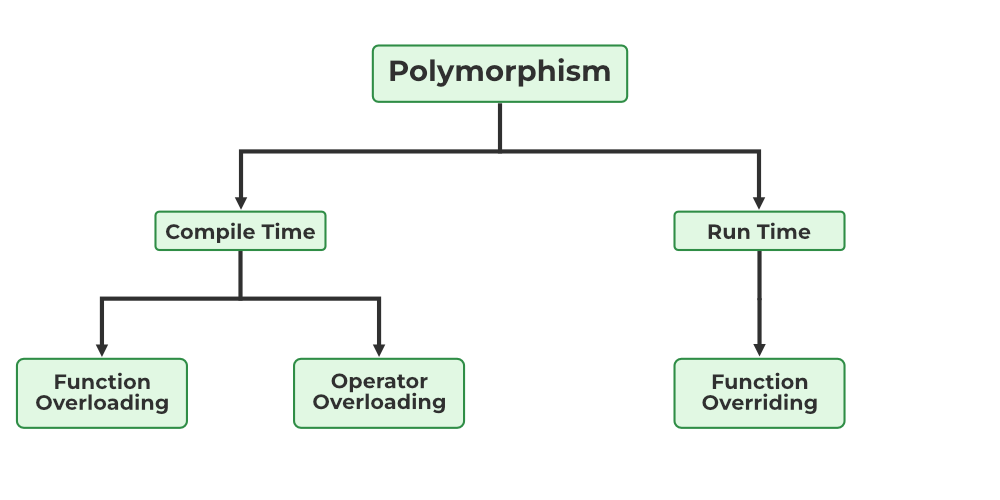Types of Polymorphism

#### A) Compile-Time Polymorphism

Compile time polymorphism, also known as static polymorphism or early binding is the type of polymorphism where the binding of the call to its code is done at the compile time. Method overloading or operator overloading are examples of compile-time polymorphism.

#### B) Runtime Polymorphism

Also known as dynamic polymorphism or late binding, runtime polymorphism is the type of polymorphism where the actual implementation of the function is determined during the runtime or execution. Method overriding is an example of this method.

Overriding is a form of runtime polymorphism where an entity with the same name but a different implementation is executed. It is implemented with the help of virtual functions.

### 17. Are there any limitations on Inheritance?

Yes, there are more challenges when you have more authority. Although inheritance is a very strong OOPs feature, it also has significant drawbacks.

• As it must pass through several classes to be implemented, inheritance takes longer to process.
• The base class and the child class, which are both engaged in inheritance, are also closely related to one another (called tightly coupled). Therefore, if changes need to be made, they may need to be made in both classes at the same time.
• Implementing inheritance might be difficult as well. Therefore, if not implemented correctly, this could result in unforeseen mistakes or inaccurate outputs.

### 18. What different types of inheritance are there?

Inheritance can be classified into 5 types which are as follows: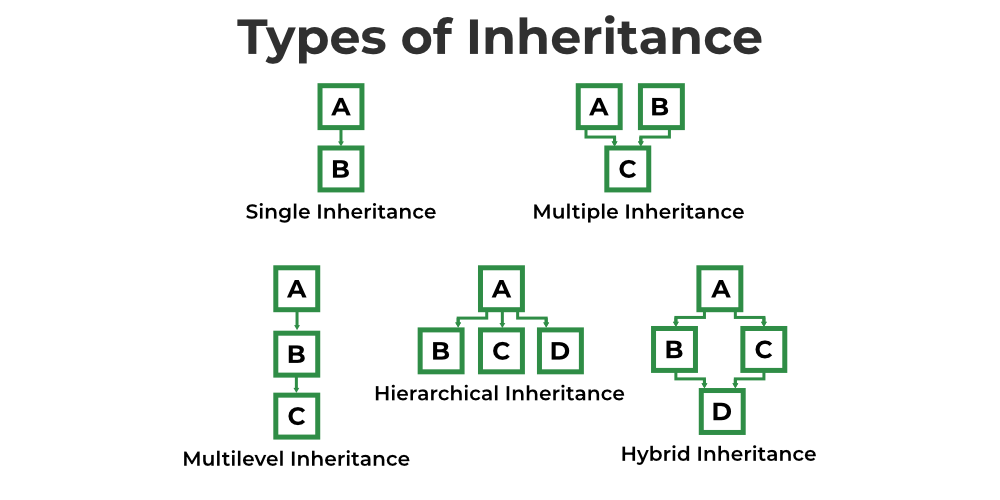1. Single Inheritance: Child class derived directly from the base class
2. Multiple Inheritance: Child class derived from multiple base classes.
3. Multilevel Inheritance: Child class derived from the class which is also derived from another base class.
4. Hierarchical Inheritance: Multiple child classes derived from a single base class.
5. Hybrid Inheritance: Inheritance consisting of multiple inheritance types of the above specified.

## Java

 `// an example of single inheritance ` `class` `Father { ` `  ``// any properties and function specific to father ` `} ` `class` `Son ``extends` `Father { ` `  ``//inherits the properties and functions of father ` `}`

## Java

 `// an example of hierarchial inheritance ` `class` `Father { ` `  ``// any properties and function specific to father ` `} ` `class` `Son ``extends` `Father { ` `  ``//inherits the properties and functions of father ` `} ` `class` `Daughter ``extends` `Father { ` `  ``//inherits the properties and functions of father ` `} `

## Java

 `// an example of multilevel inheritance ` `class` `Father { ` `  ``// any properties and function specific to father ` `} ` `class` `Son ``extends` `Father { ` `  ``//inherits the properties and functions of father ` `} ` `class` `GrandChild ``extends` `Son { ` `   `  `} `

### 19. What is an interface?

A unique class type known as an interface contains methods but not their definitions. Inside an interface, only method declaration is permitted. You cannot make objects using an interface. Instead, you must put that interface into use and specify the procedures for doing so.

### 20. How is an abstract class different from an interface?

Both abstract classes and interfaces are special types of classes that just include the declaration of the methods, not their implementation. An abstract class is completely distinct from an interface, though. Following are some major differences between an abstract class and an interface.

### 21. How much memory does a class occupy?

Classes do not use memory. They merely serve as a template from which items are made. Now, objects actually initialize the class members and methods when they are created, using memory in the process.

### 22. Is it always necessary to create objects from class?

No. If the base class includes non-static methods, an object must be constructed. But no objects need to be generated if the class includes static methods. In this instance, you can use the class name to directly call those static methods.

### 23. What is the difference between a structure and a class in C++?

The structure is also a user-defined datatype in C++ similar to the class with the following differences:

• The major difference between a structure and a class is that in a structure, the members are set to public by default while in a class, members are private by default.
• The other difference is that we use struct for declaring structure and class for declaring a class in C++.

### 24. What is Constructor?

A constructor is a block of code that initializes the newly created object. A constructor resembles an instance method but it’s not a method as it doesn’t have a return type. It generally is the method having the same name as the class but in some languages, it might differ. For example:

In python, a constructor is named __init__.

In C++ and Java, the constructor is named the same as the class name.

Example:

## C++

 `class` `Student { ` `      ``String name; ` `      ``String surname; ` `      ``int` `rollNo; ` `    ``Student() ` `    ``{ ` `        ``cout<< ``"contructor is called"``; ` `    ``} ` `}`

## Java

 `class` `Student { ` `      ``String name; ` `      ``String surname; ` `      ``int` `rollNo; ` `    ``Student() ` `    ``{ ` `        ``System.out.println(``"contructor is called"``); ` `    ``} ` `}`

## Python3

 `class` `base: ` `    ``def` `__init__(``self``): ` `        ``print``(``"This is a constructor"``) `

### 25. What are the various types of constructors in C++?

The most common classification of constructors includes:

1. Default Constructor
2. Non-Parameterized Constructor
3. Parameterized Constructor
4. Copy Constructor

#### 1. Default Constructor

The default constructor is a constructor that doesn’t take any arguments. It is a non-parameterized constructor that is automatically defined by the compiler when no explicit constructor definition is provided.

It initializes the data members to their default values.

#### 2. Non-Parameterized Constructor

It is a user-defined constructor having no arguments or parameters.

Example:

## C++

 `class` `Student { ` `      ``String name; ` `      ``String surname; ` `      ``int` `rollNo; ` `    ``Student() ` `    ``{ ` `        ``cout << ``"Non-parameterized contructor is called"` `; ` `    ``} ` `}`

## Java

 `class` `Student { ` `      ``String name; ` `      ``String surname; ` `      ``int` `rollNo; ` `    ``Student() ` `    ``{ ` `        ``System.out.println(``"Non-parameterized contructor is called"``); ` `    ``} ` `}`

## Python3

 `class` `base: ` `    ``def` `__init__(``self``): ` `        ``print``(``"This is a non-parameterized constructor"``) `

#### 3. Parameterized Constructor

The constructors that take some arguments are known as parameterized constructors.

Example:

## C++

 `class` `Student { ` `      ``String name; ` `      ``String surname; ` `      ``int` `rollNo; ` `    ``Student(String studentName, String studentSurname, ``int` `studentRollNo) ` `    ``{ ` `        ``cout << ``"Constructor with argument is called"``; ` `    ``} ` `}`

## Java

 `class` `Student { ` `      ``String name; ` `      ``String surname; ` `      ``int` `rollNo; ` `    ``Student(String studentName, String studentSurname, ``int` `studentRollNo) ` `    ``{ ` `        ``System.out.println(``"Constructor with argument is called"``); ` `    ``} ` `}`

## Python3

 `class` `base: ` `    ``def` `__init__(``self``, a): ` `        ``print``(``"Constructor with argument: {}"``.``format``(a)) `

#### 4. Copy Constructor

A copy constructor is a member function that initializes an object using another object of the same class.

Example:

## C++

 `class` `Student { ` `    ``String name, surname; ``int` `rollNo; ` `    ``Student(Student& student) ``// copy constructor ` `    ``{ ` `        ``name = student.name; ` `        ``surname=student.surname; ` `          ``rollNo= student.rollNo; ` `    ``} ` `}`

## Java

 `class` `Student { ` `    ``String name, surname; ``int` `rollNo; ` `    ``Student(Student student) ``// copy constructor ` `    ``{ ` `        ``this``.name = student.name; ` `        ``this``.surname=student.surname; ` `          ``this``.rollNo= student.rollNo; ` `    ``} ` `}`

In Python, we do not have built-in copy constructors like Java and C++ but we can make a workaround using different methods.

### 26. What is a destructor?

A destructor is a method that is automatically called when the object is made of scope or destroyed.

In C++, the destructor name is also the same as the class name but with the (~) tilde symbol as the prefix.

In Python, the destructor is named __del__.

Example:

## C++

 `class` `base { ` `public``: ` `    ``~base() { cout << ``"This is a destructor"``; } ` `}`

## Python3

 `class` `base: ` `    ``def` `__del__(``self``): ` `        ``print``(``"This is destructor"``)`

In Java, the garbage collector automatically deletes the useless objects so there is no concept of destructor in Java. We could have used finalize() method as a workaround for the java destructor but it is also deprecated since Java 9.

### 27. Can we overload the constructor in a class?

We can overload the constructor in a class. In fact, the default constructor, parameterized constructor, and copy constructor are the overloaded forms of the constructor.

### 28. Can we overload the destructor in a class?

No. A destructor cannot be overloaded in a class. The can only be one destructor present in a class.

### 29. What is the virtual function?

A virtual function is a function that is used to override a method of the parent class in the derived class. It is used to provide abstraction in a class.

In C++, a virtual function is declared using the virtual keyword,

In Java, every public, non-static, and non-final method is a virtual function.

Python methods are always virtual.

Example:

## C++

 `class` `base { ` `    ``virtual` `void` `print() ` `    ``{ ` `        ``cout << ``"This is a virtual function"``; ` `    ``} ` `}`

## Java

 `class` `base { ` `    ``void` `func() ` `    ``{ ` `        ``System.out.printIn(``"This is a virtual function"``) ` `    ``} ` `}`

## Python3

 `class` `base: ` `    ``def` `func(``self``): ` `        ``print``(``"This is a virtual function"``) `

### 30. What is pure virtual function?

A pure virtual function, also known as an abstract function is a member function that doesn’t contain any statements. This function is defined in the derived class if needed.

Example:

## C++

 `class` `base { ` `    ``virtual` `void` `pureVirFunc() = 0; ` `}`

## Java

 `abstract` `class` `base { ` `    ``abstract` `void` `prVirFunc(); ` `}`

In Python, we achieve this using @abstractmethod from the ABC (Abstract Base Class) module.

## Bonus Question

### What is an abstract class?

In general terms, an abstract class is a class that is intended to be used for inheritance. It cannot be instantiated. An abstract class can consist of both abstract and non-abstract methods.

In C++, an abstract class is a class that contains at least one pure virtual function.

In Java, an abstract class is declared with an abstract keyword.

Example:

## Java

 `abstract` `class` `Animal { ` `    ``abstract` `void` `walk(); ` `    ``void` `eat() ` `    ``{ ` `        ``System.out.println(``"The animal is eating."``); ` `    ``} ` `    ``Animal() ` `    ``{ ` `        ``System.out.println( ` `            ``"An Animal is going to be created."``); ` `    ``} ` `} ` ` `  `class` `Cow ``extends` `Animal { ` `    ``Cow() { System.out.println(``"You have created a Cow"``); } ` `    ``void` `walk() { System.out.println(``"Cow is walking."``); } ` `} ` ` `  `class` `Goat ``extends` `Animal { ` `    ``Goat() ` `    ``{ ` `        ``System.out.println(``"You have created a Goat"``); ` `    ``} ` `    ``void` `walk() { System.out.println(``"Goat is walking."``); } ` `} ` ` `  `public` `class` `OOPS { ` `    ``public` `static` `void` `main(String args[]) ` `    ``{ ` `        ``Cow cow = ``new` `Cow(); ` `        ``cow.walk(); ` `        ``cow.eat(); ` `        ``Goat goat = ``new` `Goat(); ` `        ``goat.walk(); ` `        ``goat.eat(); ` `    ``} ` `}`

In Python, we use ABC (Abstract Base Class) module to create an abstract class.

Must Refer:

Unlock the Power of Placement Preparation!
Feeling lost in OS, DBMS, CN, SQL, and DSA chaos? Our Complete Interview Preparation Course is the ultimate guide to conquer placements. Trusted by over 100,000+ geeks, this course is your roadmap to interview triumph.